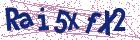# The variational iteration method for solving linear and nonlinear Schrodinger equations

Document Type : Research Article

Authors

Abstract

In this paper, the variational iteration method proposed by Ji-Huan He is applied to solve both linear and nonlinear Schrodinger equations. The main property of the method is in its flexibility and ability to solve linear and nonlinear equations accurately and conveniently. In this method, general lagrange multipliers are introduced to construct correction functionals to the problems. The multipliers in the functionals can be identified optimally via the variational theory. Numerical results show that this method can readily be implemented with excellent accuracy to linear and nonlinear Schrodinger equations. This technique can be extended to higher dimensions linear and nonlinear Schrodinger equations without a series difficulty.

Keywords20.1001.1.24236977.2009.2.1.4.6